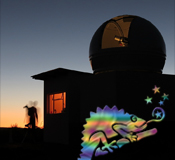CHAMÄLEON + ONJALA OBSERVATORY Special Projects SITEMAPHOME CHAMÄLEON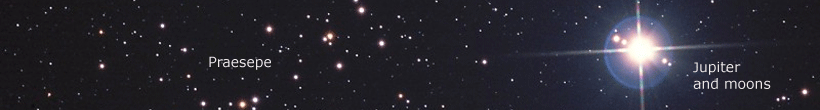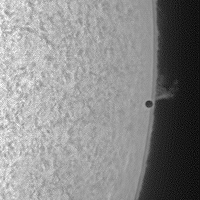Special Projects The transit of the Planet Mercury On May 9, 2016 a rare transit of Mercury in front oft the Sun took place. At time of the transit Mercury's distance to Earth was about 83,371,000 kilometers and it was seen as a small disk with a diameter of 12.1 arcseconds. « The left image (11:14:09 UTC) shows Mercury at the ingress. Back to Special ProjectsImages - SUN Images - MOON Images - SOLAR SYSTEM Images - DEEPSKY Images - WIDEFIELD Images - MISCELLANEOUS Images - SPEC. PROJECTS

May 9, 2016 - A transit of Mercury and the attempt on simultaneous observations from Namibia and Germany to derive the parallax of Mercury and thus the distance from the Sun and the value of the astronomical unit.

In principle, a Mercury or Venus transit in front of the solar disk (albeit very rarely) is rather a boring affair, although the observers can practically see the dynamics of the solar system. The animation on the right shows 5 stages of the beginning transit from 11:14 to 11:19 UTC, taken in Namibia (orientation: east left, south top). The used telescope was a 75mm Pentax refractor with 500 mm focal length, fitted with a 60mm Coronado H-alpha filter with a half-width of about 0.7 angstroms. The camera was a Celestron video module SkyRis 445 mono (stacking of 12% out of 1200 frames).

» The image at right beautifully shows the solar chromosphere. Compared to Mercury (nearly 4880 km diameter = 7.12 arcsec) you can clearly see how thin the chromosphere is in relation to the solar diameter. Also clearly visible that Mercury has missed the covering of a small protuberance at the ingress by some arcseconds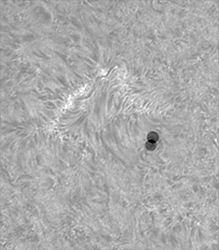To capture not only the usual photo series of transit, we had thought about whether it is possible to measure the parallax of Mercury and then to compute the solar distance and the fundamental value of the astronomical unit with amateur equipment and image processing tools.Therefore we agreed with a friend, Dirk Lucius in Ostfriesland, to take avifiles every 15 minutes at the same time with similar equipment. « The left picture shows the parallax of Mercury, based on the solar chromosphere, at exactly 14:00:15 UTC (midtime of the Avifiles) between Leer / Ostfriesland and Onjala Lodge in Namibia. The focal lengths were about 1.000 millimeters. The displacement is approximately 3/4 of the apparent Mercury diameter. All of the following images can be enlarged by clicking on it.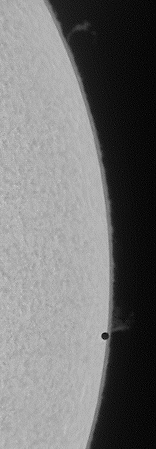The station Onjala / Namibia The station Leer / Ostfriesland Measurement of the parallax

 The Station Onjala (22 ° 12 ' 24.2'' south, 17 ° 34 ' 58.8 " east, height about 1724 m above sea level)To get optimal seeing conditions we put the telescope on the transportable mount and not in the dome. The 75mm Pentax refractor with 500mm focal length was set up parallel to a fixed mounted C11 with HyperStar so that the objective of the C11 pointed to the ground and the main mirror cell of the C11 was covered with rescue foil to minimize thermal gradients.A Celestron video module Skyris 445 M with a pixel size of 3.75microns was used for capturing the avifiles. Each avifile had a length of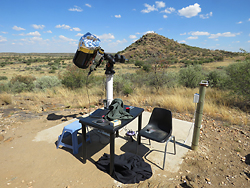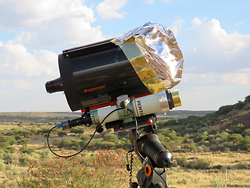700 frames, of which 20% have been stacked. The computer clock was set with an accuracy of below 1 second with the help of a GPS handheld receiver. It can be clearly seen in the above images that the weather conditions in Namibia were far from optimal. We had during our whole stay on Onjala nearly every day the same situation: in the morning was a clear blue sky and from noon rising convective clouds and in the evening a mostly complete covered sky.

Due to the increasing clouds we could significantly take less Avifiles than we had planned. Overall, we had finally only six simultaneous recordings for a further analysis.

For measureing the parallax of Mercury we needed as many details and fine structures on the solar disk as possible. So we took H-alpha images of the solar chromosphere. On Onjala a 60mm Coronado (HWB approximately 0.7 Ångström) and in Ostfriesland a 2-fold stacked 90mm Coronado filter (HWB about 0.5 Angstroms) was used.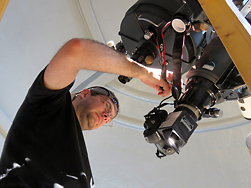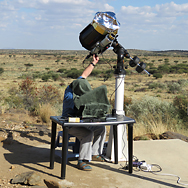While for one of us (Paech) a 4 hours sweating session under a towel (shading of the laptop screen) started, the other one of us (Hofmann) was in the 4m dome and took images at the Zeiss APQ refractor (150 / 1200mm) with a Canon 6D and an old Baader Herschel prism images in white light.In the following are some white light, H-alpha images and animations of the Mercury transit. Because of the rapidly increasing cloudiness we stopped taking images at 15:30 UTC (17:30 CEST). In Namibia the sun was setting around 16:30 UTC.
Above 4 white light images taken with the Zeiss APQ refractor, Canon 6D and an old Baader Herschel prism (image orientation: east left, north up). The two images on the left were taken shortly after the ingress. The picture on the right just before stopping our image session due to the dense cloud coverage. The images can be enlarged by clicking.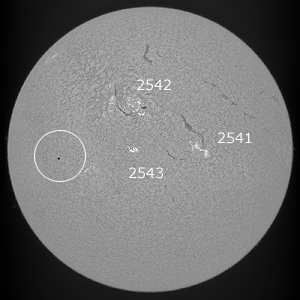To the H-alpha images of Onjala. (Click to load a large images): The left image shows the entire sun at 12:45 UTC with the three marked active regions NOAA 2541, 2542 and 2543.

Further images taken at different phases of transit showing the entire sun: 11:02 (still without Mercury), 11:31, 12:15, 13:17 and 14:51 11:02 (still without Mercury), 11:31, 12:15, 13:17 und 14:51 (all times are UTC). (all times are UTC). Below we show two animations which can be enlarged by clicking on them. left: 14:11 to 14:33 UTC, captured with f = 1.000 mm and right 11:15 to 13:50 UTC with f = 500 mm.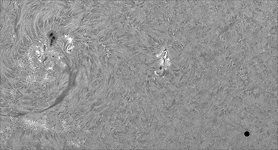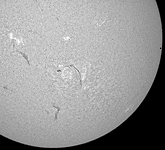Note the changing chromospheric phenomena.

 The station Leer in Ostfriesland (N 53 ° 14 '44.4 ", E 07 ° 28' 36.0", height about 3 m above sea level)In Germany Dirk Lucius watched the transit of Mercury at the same time. He used a 90mm refractor with a Coronado Double Stack H-alpha filter. The camera was the same as in Namibia - a Celestron video module SkyRis 445 M. The focal length oft he telescope has been extended with a Baader FFC module to about 1.000 mm.The image far right shows the average quality of the summed video images of Dirk. Both images can be enlarged by clicking.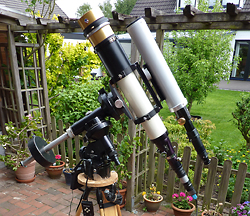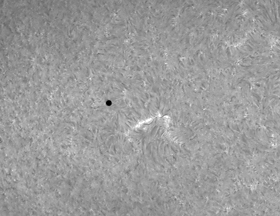In addition to the simultaneous images fort he parallax measurement Dirk was still working with its 150 mm refractor and exposed images in the three spectral ranges (from left to right) continuum (white light), the calcium and in H-alpha light with different focal lengths.

Measurement of the parallax of Mercury and the determination of the astronomical unit (AU)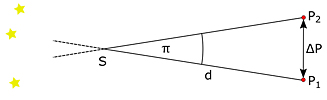Knowing on one hand the parallax of a celestial body with respect to much more distant background objects and on the other hand the distance between the two observation stations $$\Delta P$$ The distance of the celestial body can be purely geometrically determined from these two measured values. In most cases the parallax angle $$\pi$$ are very small angles in astronomy. This simplifies the calculation and the distance $$d$$ to the body S. S can be determined using the simple formula, see figure 1 on the left: $$d = \frac{\Delta P}{\pi}$$
Unfortunately, the parallax of the Sun and thus the AU can not be measured directly in the context of a quasi "infinitely" distant star. However, the observation of a transit of Mercury or Venus and the knowledge of the relative distances in the solar system, for example, based on the Kepler's third law, gives an access to the Astronomical Unit.

 The approach we use is based on the simultaneous observation of the position of Mercury in front of the Sun. The recorded images from Leer and Onjala were matched together and the remaining parallax of Mercury $$\pi'_M$$ determined with respect to the solar surface. $$\pi'_M$$ is a combination of the parallax of the sun $$\pi_S$$ and Mercury $$\pi_M$$ relative to the background stars, as shown in Figure 2 (right). In the left part, the Sun, Mercury's disc and the background stars are shown as they appear from the location 1 on the Earth. In the central part of the picture, the view of the Sun and Mercury from location 2 on the Earth is superimposed. Relative to the fixed background stars the parallax of Mercury and the Sun could be read here. But the background stars but not visible during daylight, only the parallax with respect to the Sun's surface can be determined (right part) with$$\pi'_M=\pi_M-\pi_S$$.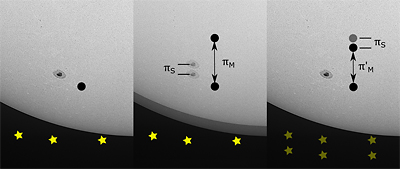From long-term observations the relative distances between Earth and Mercury $$d_{M}$$ and Earth and Sun $$d_S$$ at the transit time can be determined in multiples of AE. Since these observations are beyond our possibilities, we used the site www.calsky.com to determine these quantities. We found the factors $$d_S=1.01$$ and $$d_M=0.558$$.

The astronomical unit is designated in the following formula with x and can be computed with the quantities from above to $$x=\frac{\Delta P}{\pi'_Md_S}\left(\frac{d_s}{d_M}-1\right)$$. The derivation is described in detail in our formulary (in German) .

The determination of Mercury's parallax $$\pi'_M$$ in corresponding images will be briefly described below.

 Determination of the scale of the Onjala images with the help of the Sun's diameter of 1900.8 arcseconds (www.calsky.com) for the transit period. Matching of the images from Leer to the Onjala images based on the H-α structures. To reduce the effort for a manual referencing in Photoshop, the recordings were skaled by factor of 8 (Onjala) and 4 (Leer), 2 artificial stars were copied at prominent H-α structures (see Figure 3 on the right) and automatically aligned in the program MaximDL. Determination of the pixel coordinates of Mercury's discs in the two images for each observation time. This was done in the scaled images by creating a large circle around Mercury in Photoshop and using the function "free transformation" until the disc of Mercury was centered. The displayed pixel coordinates of the center will be used as the measured position values, see Figure 4. Conversion of the distance in pixels into the parallax $$\pi'_M$$ in the sky with help of the imaging scale and the scaling factor of the Onjala images.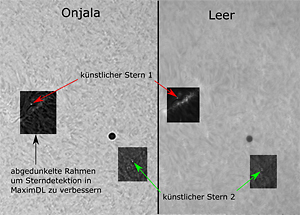Figure 3
 With the help of the station coordinates (length, width, height) of Leer and Onjala the position vectors $$\vec{p}_2$$ and $$\vec{p}_1$$ are calculated in an Earth-fixed cartesian reference system. Rotation of the two vectors in the ICRS (see below) with rotation data from the Earth Orientation Center. Determination of the vector $$\vec{g}_0$$, that is the direction to Mercury in space, with data of JPL Horizons website. In principle this vector can be determined with knowledge of the position of Mercury in the sky and the time, but here we save us this work. The reduced distance is calculated with the cross product of the vectors $$\Delta P=\left|\left(\vec{p}_2-\vec{p}_1\right)\times\vec{g}_0\right|.$$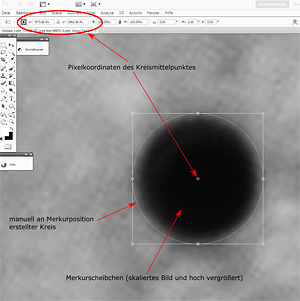Figure 4 »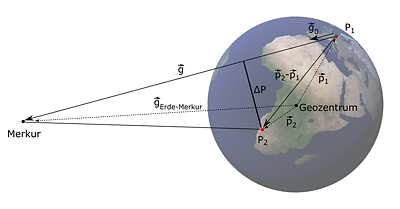The calculation of the reduced distance $$\Delta P$$, perpendicular to the direction from the observation location to Mercury, between Onjala and Leer is briefly summarized here. The necessary steps and formulas are listed in the formulary. The basis, considered separately for each observation time, are spatial triangles between Merkur - Onjala ($$P_2$$) - Leer ($$P_1$$). Figure 5 (left) shows an example triangle and the vectors used therein. For the calculation it is important that all vectors are present in a common coordinate system. Here the independently of the Earth's rotation celestial reference system ICRS is useful. « Figure 5

The table lists the results of the parallax of Mercury $$\pi'_M$$, related to the solar surface, the reduced distance $$\Delta P$$ between Onjala and Leer and the calculated values for the astronomical unit $$x$$ for each measurement time. The mean value is 149,931,751 km with a standard deviation of 5.6 million km

Measuring time [UTC] $$\pi'_M$$ ["] $$\Delta P$$ [km] $$x$$ [km]

11:15:12.5 8.516 7807.17 151 657 264
12:30:12.5 8.936 7782.32 144 068 962
13:00:12.5 8.263 7761.36 155 383 158
13:15:12.5 9.044 7747.67 141 722 132
13:45:12.5 8.263 7712.88 154 413 326
14:00:12.5 8.352 7691.46 152 345 665

The animation on the right shows the parallaxe with respect to the solar chromosphere between Leer and Onjala at 14:00:00 UTC »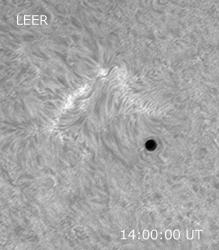At the beginning of this project we had not believed that it is possible to determine the astronomical unit so accurately with the used small instruments. The difference to the SI value of the astronomical unit is on average just 334,000 km, i.e. slightly less than the average distance between Earth and Moon.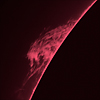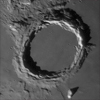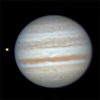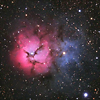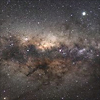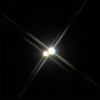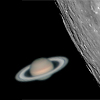Sun Moon Solar System DeepSky Widefield Miscellaneous Spec. Projects

All Images and all Content on this page are © by Franz Hofmann, Wolfgang Paech und Dirk Lucius